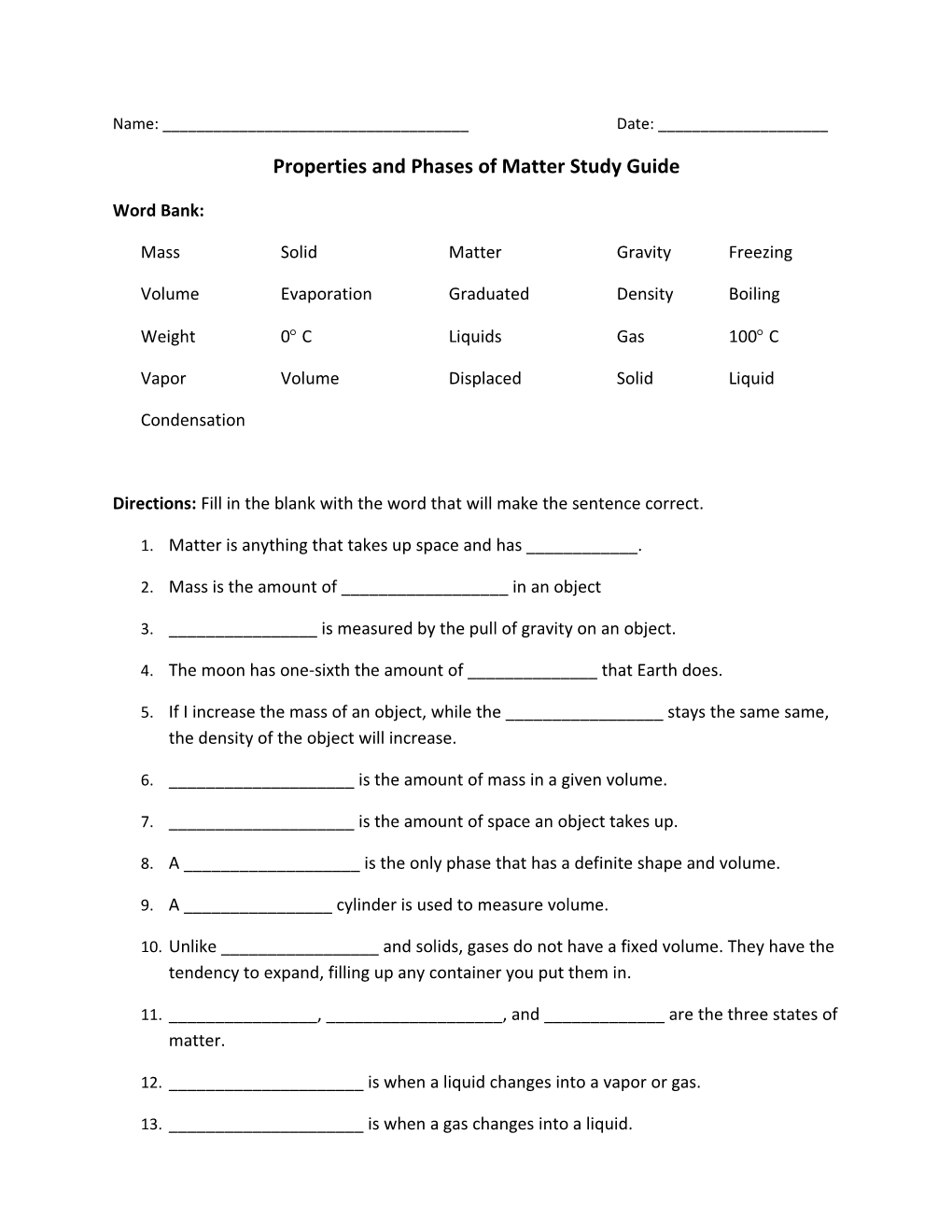# Properties and Phases of Matter Study GuideName: ______Date: ______

Properties and Phases of Matter Study Guide

Word Bank:

MassSolidMatterGravityFreezing

Weight0 CLiquidsGas100 C

VaporVolumeDisplacedSolidLiquid

Condensation

Directions: Fill in the blank with the word that will make the sentence correct.

1. Matter is anything that takes up space and has ______.
2. Mass is the amount of ______in an object
3. ______is measured by the pull of gravity on an object.
4. The moon has one-sixth the amount of ______that Earth does.
5. If I increase the mass of an object, whilethe______stays the same same, the density of the object will increase.
6. ______is the amount of mass in a given volume.
7. ______is the amount of space an object takes up.
8. A ______is the only phase that has a definite shape and volume.
9. A ______cylinder is used to measure volume.
10. Unlike ______and solids, gases do not have a fixed volume. They have the tendency to expand, filling up any container you put them in.
11. ______, ______, and ______are the three states of matter.
12. ______is when a liquid changes into a vapor or gas.
13. ______is when a gas changes into a liquid.
14. The ______point is where the temperature reaches ______and liquid changes into a solid.
15. The boiling point is where the temperatures reaches ______and water evaporates into water ______.
16. We can measure the volume of an object by calculating how much water the object ______.
1. Explain IN DETAIL using SCIENTIFIC VOCABULARY how adding heat or taking it away can affect the state of matter it’s in.

______

Draw a picture to illustrate what you described above.

1. What is the difference between mass and weight?

______

1. Calculate the volume of a carrot if the water in the graduated cylinder was 154 mL before the carrot was put in and the volume of the liquid after the carrat was submerged rose to 186mL. SHOW YOUR WORK!
1. Write the three formulas for finding mass, volume, and density:
2. Mass =
3. Volume =
4. Density =
5. What is the second formula for finding the volume of a regular shape?
6. Volume =
1. What is the label for mass? ______
2. What is the label for volume? ______or ______
3. What is the label for density? ____/______or _____/______
4. What is the density of water? (LABEL!!) ______
5. Give an example of an object’s density that would:
6. FLOAT in water: ______(LABEL!!!)
7. SINK in water: ______(LABEL!!!)
1. Mass = 16g, Volume = 22 mL, Find the DENSITY (Round to the nearest hundredth and LABEL!)
1. Will the object float? How do you know?
1. Volume = 45 mL, Density= 0.56, Find the MASS (Round to the nearest hundredth and LABEL!)
1. Why do solids have a fixed shape while liquids and gases do not? ______